Courses

# Integral Theorems Civil Engineering (CE) Notes | EduRev

## Civil Engineering (CE): Integral Theorems Civil Engineering (CE) Notes | EduRev

The document Integral Theorems Civil Engineering (CE) Notes | EduRev is a part of Civil Engineering (CE) category.
All you need of Civil Engineering (CE) at this link: Civil Engineering (CE)

Divergence Theorem
Suppose u(x) is a vector field defined on some convex three dimensional region, R in physical space with volume v and on a closed surface a bounding this volume and u(x) is continuous in R and continuously differentiable in the interior of R. Then,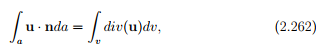where, n is the outward unit normal field acting along the surface a, dv and da are infinitesimal volume and surface element, respectively. Proof of this theorem is beyond the scope of these notes.
Using (2.262) the following identities can be established: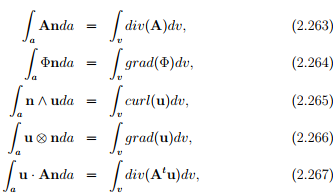where Φ, u, A are continuously differentiable scalar, vector and tensor fields defined in v and continuous in v and n is the outward unit normal field acting along the surface a.

Stokes theorem
Stokes theorem relates a surface integral, which is valid over any open surface a, to a line integral around the bounding single simple closed curve c in three dimensional space. Let dx denote the tangent vector to the curve, c and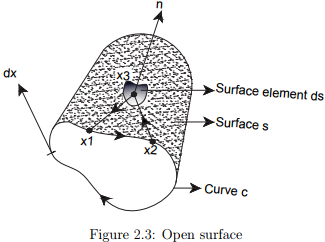denote the outward unit vector field n normal to s. The curve c has positive orientation relative to n in the sense shown in figure 2.3. The indicated circuit with the adjacent points 1, 2, 3 (1, 2 on curve c and 3 an interior point of the surface s) induced by the orientation of c is related to the direction of n (i.e., a unit vector normal to s at point 3) by right-hand screw rule. For a continuously differentiable vector field u defined on some region containing a, we have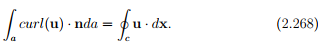Let Φ and u be continuously differentiable scalar and vector fields defined on a and c and n is the outward drawn normal to a. Then, the following can be show :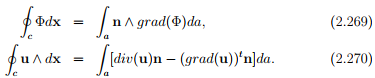Green’s theorem
Finally, we record Greens’s theorem. We do this first for simply connected domain and then for multiply connected domain. If any curve within the domain can be shrunk to a point in the domain without leaving the domain, then such a domain is said to be simply connected. A domain that is not simply connected is said to be multiply connected.

Applying Stoke’s theorem, (2.268) to a planar simply connected domain with the vector field given as u = f(x, y)e1 + g(x, y)e2,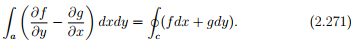The above equation (2.271) when f(x, y) = 0 reduces to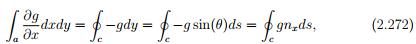where θ is the angle made by the tangent to the curve c with e1 and nis the component of the unit normal n along e1 direction, refer to figure 2.4. Then, when g(x, y) = 0 equation (2.271) yields,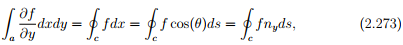where ny is the component of the unit normal along the e2 direction. Similarly, applying Stoke’s theorem (2.268) to a vector field,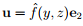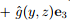defined over a planar simply connected domain,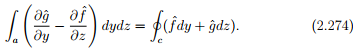The above equation (2.274) when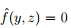reduces to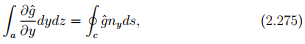where ny is the component of the normal to the curve along the e2 direction and when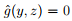yields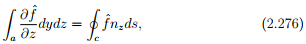where nz is the component of the normal to the curve along the e3 direction.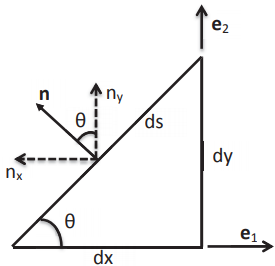Figure 2.4: Relation between tangent and normal vectors to a curve in xy plane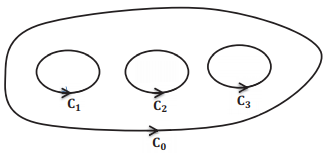Figure 2.5: Illustration of a multiply connected region

In a simply connected domain, the region of interest has a single simple closed curve. In a multiply connected domain, the region of interest has several simple closed curves, like the one shown in figure 2.5. If the line integrals are computed by traversing in the direction shown in figure 2.5,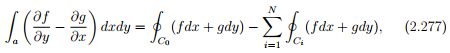where the functions f and g are continuously differentiable in the domain, C0 is the outer boundary of the domain, Ci ’s are the boundary of the voids in the interior of the domain and we have assumed that there are N such voids in the domain. We emphasize again our assumption that we transverse the interior voids in the same sense as the outer boundary.
The above equation (2.277) when f(x, y) = 0 reduces to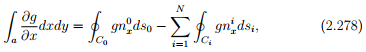where nix is the component of the unit normal n along e1 direction of the i th curve. Then, when g(x, y) = 0 equation (2.277) yields,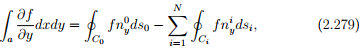where niy is the component of the unit normal along the e2 direction of the ith curve.

The document Integral Theorems Civil Engineering (CE) Notes | EduRev is a part of Civil Engineering (CE) category.
All you need of Civil Engineering (CE) at this link: Civil Engineering (CE)Use Code STAYHOME200 and get INR 200 additional OFF Use Coupon Code
All Tests, Videos & Notes of Civil Engineering (CE): Civil Engineering (CE)

### Top Courses for Civil Engineering (CE)### Top Courses for Civil Engineering (CE)Track your progress, build streaks, highlight & save important lessons and more!

,

,

,

,

,

,

,

,

,

,

,

,

,

,

,

,

,

,

,

,

,

;